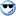Wrox Programmer Forumsif else question
 | Search | Today's Posts | Mark Forums Read
 Javascript General Javascript discussions.
 Welcome to the p2p.wrox.com Forums. You are currently viewing the Javascript section of the Wrox Programmer to Programmer discussions. This is a community of software programmers and website developers including Wrox book authors and readers. New member registration was closed in 2019. New posts were shut off and the site was archived into this static format as of October 1, 2020. If you require technical support for a Wrox book please contact http://hub.wiley.com
 hoonboon69Registered User Join Date: Aug 2003 Location: , , . Posts: 2 Thanks: 0 Thanked 0 Times in 0 Postsif else question

I'm trying to write a script that will give a certain response based upon a numeric value that is generated from values entered into a form. the user enters two numeric values(V1 and V2) and clicks a button that tells you the percentage that V1 is of V2. if that percentage is less than say.....80, than a certain message is generated ELSE another answer is generated. i've been able to write the calculator part, but am having problems with the subsequent statement. my code is as follows....

<html>
<SCRIPT language=JavaScript>
<!--

function tom1() {
a = document.form1.c.value;
b = document.form1.d.value;
c = a/b;
d = c*100;
document.form1.total2.value = d
}
//-->
</SCRIPT>
<FORM name=form1>
<TBODY>
<TR>
</TD></TR>
<TR><TD><INPUT size=5 name=c> is what percent of <INPUT size=5
name=d>?</TD>
<TD><INPUT onclick=tom1() type=button value=Calculate></TD></TR>
<TR>
<TD align=middle
colSpan=3><INPUT type=reset value=Reset></TD></TR></TBODY></TABLE></FORM>
<SCRIPT Language=JavaScript>

<!-- This part isn't working

if (document.form1.Calculate < 80) {
document.write("no")
}
else {
document.write("yes")
}
//-->
</SCRIPT>
</body>
</html>

any suggestions would be appreciated

 MoharoFriend of Wrox Join Date: Jun 2003 Location: , , USA. Posts: 101 Thanks: 0 Thanked 1 Time in 1 Posterror you get is because the 'if' statement is incorrect. your answer field is named in your code 'total2' so why are you writing "calculate". besides if you want to refer to a text field you gotta have 'name' or 'id' attribute for the <input> element. don't forget about the 'type' attribute either. if you want to check whether your answer is greater than 80 or less, you have to do it by invoking the function because when your browser window loads, you have your form elements empty so javascript can't tell if the result is greater or less than 80. just check out how i rewrote the code...

<html>

<body>

<SCRIPT language=JavaScript>
<!--

function getPercentage() {
a = parseFloat(document.myform.c.value); //make sure that variable 'a' is an number
b = parseFloat(document.myform.d.value); //same here
c = a/b;
d = c*100;
document.myform.total.value = d;
else if(d>=70 && d<=80) answer.innerHTML = "answer is between 70% and 80%";
else if(d>=60 && d<=70) answer.innerHTML = "answer is between 60% and 70%";
else answer.innerHTML = "is somewhere between 0% and 60%";
}
//-->
</SCRIPT>

<FORM name="myform">
<TBODY>
<TR>
<TD align=middle colSpan=3>
</TD>
</TR>
<TR>
<TD>
<INPUT type="text" size=5 name="c"> is what percent of
<INPUT type="text" size=5 name="d">?
</TD>
<TD>Answer: <INPUT type="text" size=5 name="total"> %
</TD>
<TD>
<INPUT onclick="getPercentage();" type=button value="Calculate" name="calc">
</TD>
</TR>
<TR>
<TD align=middle colSpan=3>
<INPUT type=reset value=Reset>
</TD>
</TR></TBODY></TABLE></FORM>

</span>

</body>
</html>

:D:D:D:D:):)the genuine geniusSimilar Threads Thread Thread Starter Forum Replies Last Post question maheshraju ASP.NET 2.0 Basics 3 March 13th, 2008 08:54 AM Question Ashwini Classic ASP Databases 3 January 10th, 2006 07:20 AM Question? Calibus Classic ASP Databases 8 August 6th, 2004 08:25 AM a question gorji C++ Programming 2 August 11th, 2003 07:41 AMCopyright (c) 2020 John Wiley & Sons, Inc.